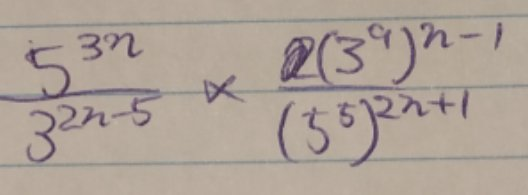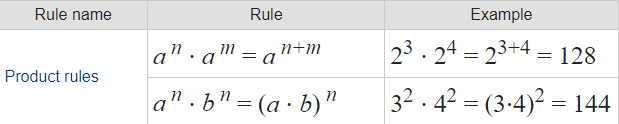Can't figure out how to solve this!

Pedja

New member
$\frac{2^{5x}}{32^x}=\frac{2^{5x}}{(2^5)^x}=\frac{2^{5x}}{2^{5x}}=2^{5x-5x}=2^0=1$
Your exercises could be done in a similar way.

•Otis

Dr.Peterson

Elite Member
Hi, I would like to get some help on question d.

I don't even know where to startYou'll do the same sort of thing you did for (c), only more so. If nothing else, show us your work for (c), so we can use that as a start.

As you presumably did there, you can start by expressing some of the bases as powers of other bases. After all, that's the hint they gave you!

Otis

Elite Member
$= \frac{2^{5x}}{2^{5x}} = 2^{5x-5x} = 2^0 = 1$
I like your example, yet I would have finished with

$= \frac{2^{5x}}{2^{5x}} = 1$Otis

Elite MemberThat's good, Rengoku, with two fixes.

3^9 = 19683 not 27

5^5 = 3125 not 125

Next, use the commutative property to write a ratio of powers of 3 multiplied by a ratio of powers of 5. In other words, swap the numerators. Then, expand the exponents (use distributive property).

See whether that helps you finish.Harry_the_cat

Elite Member
The best thing you can do with these sort of questions is "get to know" the powers of 2, 3 and 5.
ie, $$\displaystyle 2^0=1, 2^1=1, 2^2=4, 2^3=8, 2^4=16, 2^5= 32, 2^6=64, 2^7=128, 2^8=256, 2^9=512, 2^{10}=1024$$
$$\displaystyle 3^0=1, 3^1=3, 3^2=9, 3^3=27, 3^4=81, 3^5=243$$
$$\displaystyle 5^0=1, 5^2=25, 5^3=125, 5^4=625$$ for starters.

The first "thing" I see when looking at (d) is that all the bases can be written in terms of 3s and 5s. That's because I know that $$\displaystyle 3^3=27$$, and $$\displaystyle 5^3=125$$. If you don't know those basic facts, these questions are so much more difficult. Take the time to make those numbers your friends!

I used to tell my students to write the above on a big piece of paper, paste it up in the toilet, and look at it every day!!

So the first thing to do is replace 27 with ($$\displaystyle 3^3)$$ and 125 with ($$\displaystyle 5^3)$$ which is what you attempted to do. Brackets are very important.

Now show us what you can do.

•Jomo, Otis and Subhotosh Khan

Rengoku0510

New member
After spending all afternoon I finally managed to get the right answerThank you so much everyone!!!

I'll rest my brain for now and try solving this again tomorrow to see if I could do it without getting stuck!

•Otis and Harry_the_cat

Harry_the_cat

Elite Member
After spending all afternoon I finally managed to get the right answerThank you so much everyone!!!

I'll rest my brain for now and try solving this again tomorrow to see if I could do it without getting stuck!
Good on you for not giving up!! Well done!

New member
May I post my attempt at this since op has said they have got the answer?

•mmm4444bot

mmm4444bot

Super Moderator
Staff member
May I post my attempt at this since op has said they have got the answer?
Yes, that's fine.Harry_the_cat

Elite Member
You were correct right up until the last step. You can't do that. You are saying something like $$\displaystyle 2^4 * 3^5 = 6^9$$. That's not correct is it?

lookagain

Elite Member
Sorry for poor quality... Last line says: 5^- 3n-3 * 3^n+2 = 15^-2n-1

When you type this in horizontal style, you must use grouping symbols, as in
5^(-3n - 3)*3^(n + 2).

New member
You were correct right up until the last step. You can't do that. You are saying something like $$\displaystyle 2^4 * 3^5 = 6^9$$. That's not correct is it?So the first rule doesn't apply here since they don't have the same base, thanks.

I'm not sure if the second rule applies; does the fact that they both have n as part of their exponents mean they have the same exponents or must it be exactly 3n-3 or n+2?

EDIT:

Question says simplify so do I just leave it as 5^(-3n - 3)*3^(n + 2)? Is it not possible to multiply these then?

Last edited:

Harry_the_cat

Elite Member
The second rule doesn't apply either because the exponents are not the same.
The answer is the LHS of your last line. You can't do anything more. To simplify the expression. It's important to know when to stop.

New member
Thanks very very much!

lookagain

Elite Member
Question says simplify so do I just leave it as 5^(-3n - 3)*3^(n + 2)? Is it not possible to multiply these then?
The answer is the LHS of your last line. You can't do anything more. To simplify the expression. It's important to know when to stop.

There is an alternative. Write it as the simplified fraction

$$\displaystyle \dfrac{3^{n + 2}}{5^{3n + 3}}.$$

Last edited:
•Otis

New member
There is an alternative. Write it as the simplified fraction

$$\displaystyle \dfrac{3^{n + 2}}{5^{3n + 3}}.$$
Thanks but how do you know 3^(n+2) is the numerator and not 5^(3n+3)?

lookagain

Elite Member
Thanks but how do you know 3^(n+2) is the numerator and not 5^(3n+3)?

If you have a positive leading coefficient for the exponent, then the corresponding term goes in
the numerator and vice versa.

See that 5^(-3n - 3) from the product is equal to
5^[-(3n + 3)]. This is equivalent to 1/[5^(3n + 3)].

I must go now.

•